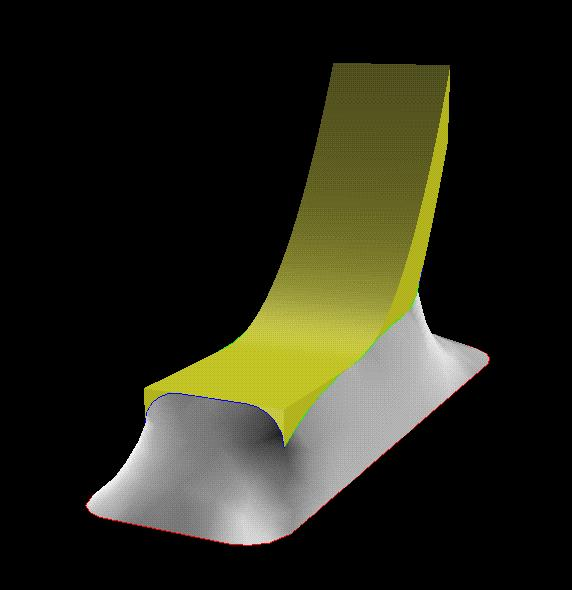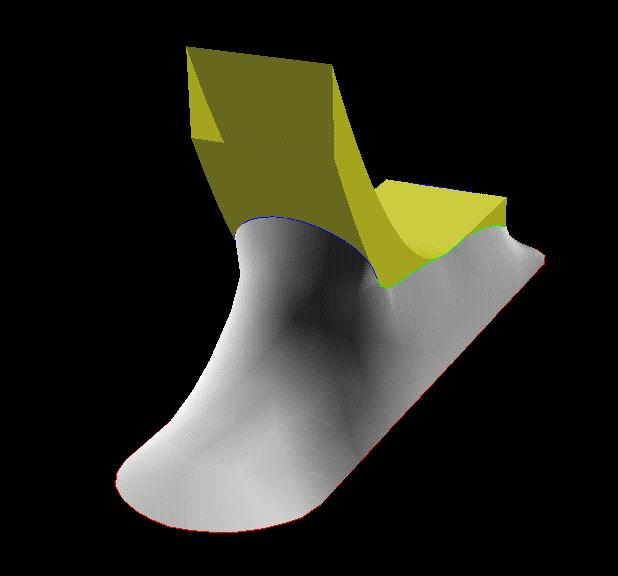The Gull Wing Solder Joint

This file models the geometry of a gull wing solder joint. The geometry of the lead is determined by connecting 3 parabolas. The user is required to input various fitting parameters, geometric parameters for the dimensions of the lead, and materials parameters for the solder.

Contact angle on sides of the gull wing (degrees):
Contact angle on the bottom surface of the gull wing (degrees):
Contact angle on the toe of the gull wing (degrees):
Wetting angle on the pad (degrees):
Thickness of lead in the z direction. (mils where 1 mil = 0.001 inches):
Width of lead in y direction (mils):
Width of the pad in the y direction (mils):
Volume of the solder (cubic mils):
This parameter can be used to change the initial conditions of the surface:
This parameter can be used to change the initial conditions of the surface:
Used to determine the magnitude of the perturbation in the force calculation:
Liquid-vapor surface energy of solder (Kg/s^2):
Density of solder (Kg/mil^3):
Height of the bottom of the gull foot above the pad surface.:
Toe of gull wing (start of zero slope section):
End of zero slope section of gull wing:
An x coordinate on the first parabola:
A y coordinate on the first parabola:
Parabola joining point (The slopes of the parabolas match here.):
An x coordinate on the second parabola:
A y coordinate on the second parabola: